# NCERT Solutions for Class 9 Maths Exercise 10.3## myCBSEguide App

CBSE, NCERT, JEE Main, NEET-UG, NDA, Exam Papers, Question Bank, NCERT Solutions, Exemplars, Revision Notes, Free Videos, MCQ Tests & more.

NCERT solutions for Class 9 Maths Circles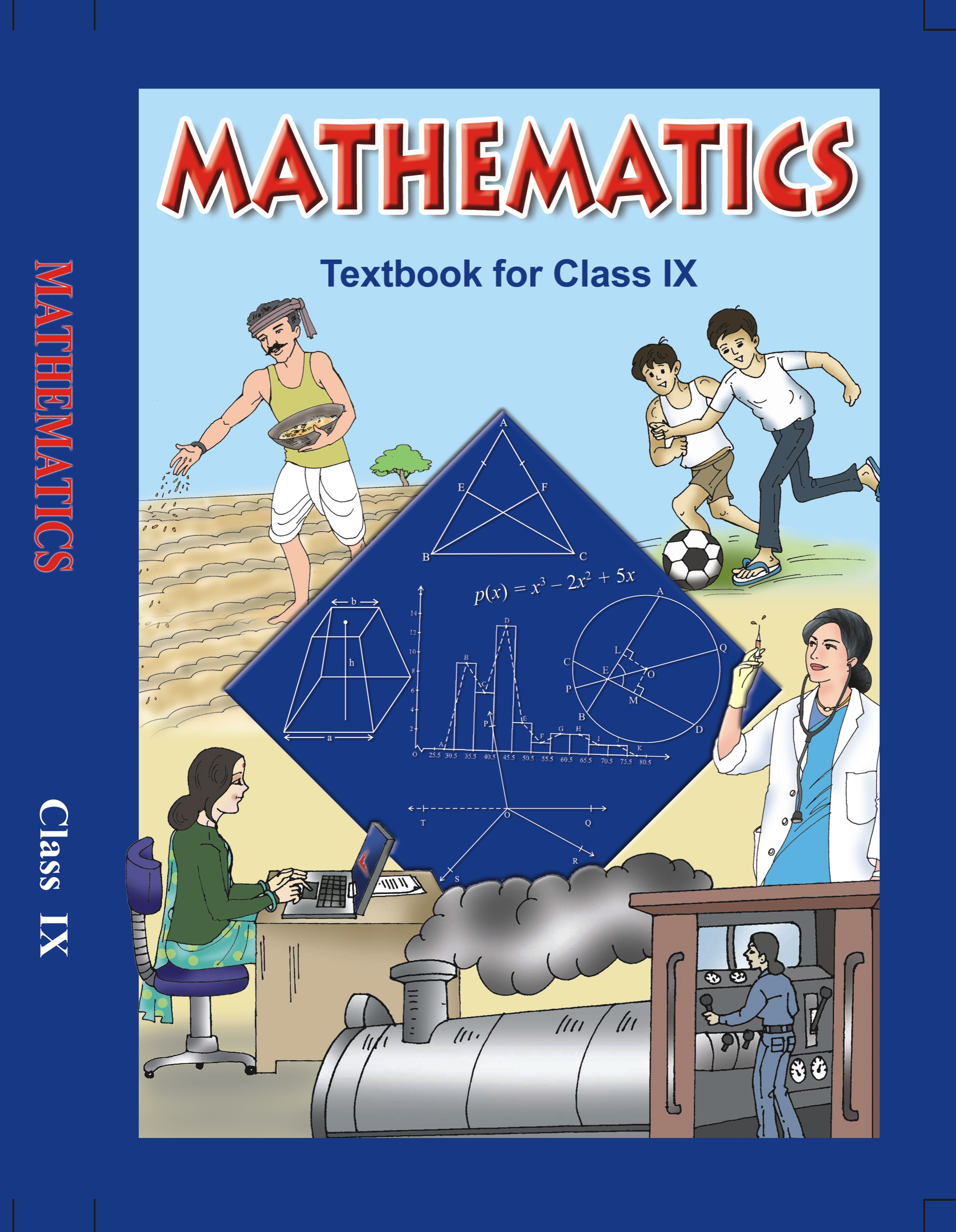## NCERT Solutions for Class 9 Mathematics Circles

###### 1. Draw different pairs of circles. How many points does each pair have in common? What is the maximum number of common points?

Ans. From the figure, we observe that when different pairs of circles are drawn, each pair have two points (say A and B) in common. Maximum number of common points are two in number.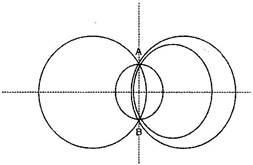Suppose two circles C (O,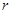) and C (O’,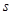) intersect each other in three points, say A, B and C.

Then A, B and C are non-collinear points.

We know that:

There is one and only one circle passing through three non-collinear points.

Therefore, a unique circle passes through A, B and C.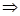O’ coincides with O and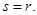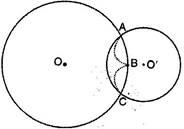A contradiction to the fact that C (O’,)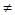C (O,)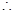Our supposition is wrong.

Hence two different circles cannot intersect each other at more than two points.

NCERT Solutions for Class 9 Maths Exercise 10.3

###### 2. Suppose you are given a circle. Give a construction to find its centre.

Ans. Steps of construction:

(a) Take any three points A, B and C on the circle.

(b) Join AB and BC.

(c) Draw perpendicular bisector say LM of AB.

(d) Draw perpendicular bisector PQ of BC.

(e) Let LM and PQ intersect at the point O.

Then O is the centre of the circle.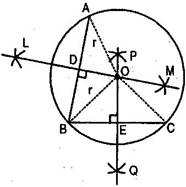Verification:

O lies on the perpendicular bisector of AB.OA = OB ……….(i)

O lies on the perpendicular bisector of BC.OB = OC ……….(ii)

From eq. (i) and (ii), we observe that

OA = OB = OC =(say)

Three non-collinear points A, B and C are at equal distance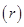from the point O inside the circle.

Hence O is the centre of the circle.

NCERT Solutions for Class 9 Maths Exercise 10.3

###### 3. If two circles intersect at two points, prove that their centres lie on the perpendicular bisector of the common chord.

Ans. Given: Let C (O,) and C (O’,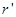) be two circles intersecting at A and B. AB is the common chord.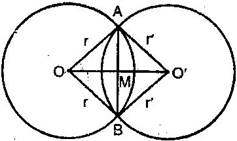To prove: OO’ is the perpendicular bisector of the chord AB.

Construction: Join OA, OB, O’A, O’B.

Proof: In triangles OAO’ and OBO’,

OO’ = OO’ [Common]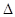OAO’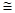OBO’ [By SSS congruency]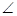AOO’ =BOO’ [By CPCT]AOM =BOM

Now inAOB, OA = OB

AndAOB =OBA [Proved earlier]

AlsoAOM =BOMRemainingAMO =BMOAMO =BMO =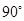[Linear pair]OM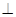ABOO’AB

Since OMABM is the mid-point of AB.

Hence OO’ is the perpendicular bisector of AB.

## NCERT Solutions for Class 9 Maths Exercise 10.3

NCERT Solutions for Class 9 Maths PDF (Download) Free from myCBSEguide app and myCBSEguide website. Ncert solution class 9 Maths includes text book solutions from Mathematics Book. NCERT Solutions for CBSE Class 9 Maths have total 15 chapters. 9 Maths NCERT Solutions in PDF for free Download on our website. Ncert Maths class 9 solutions PDF and Maths ncert class 9 PDF solutions with latest modifications and as per the latest CBSE syllabus are only available in myCBSEguide.

## CBSE app for Class 9

To download NCERT Solutions for Class 9 Maths, Computer Science, Home Science,Hindi ,English, Social Science do check myCBSEguide app or website. myCBSEguide provides sample papers with solution, test papers for chapter-wise practice, NCERT solutions, NCERT Exemplar solutions, quick revision notes for ready reference, CBSE guess papers and CBSE important question papers. Sample Paper all are made available through the best app for CBSE students and myCBSEguide website.### 3 thoughts on “NCERT Solutions for Class 9 Maths Exercise 10.3”

1. AOB = OBA in ques 3 is written wrong